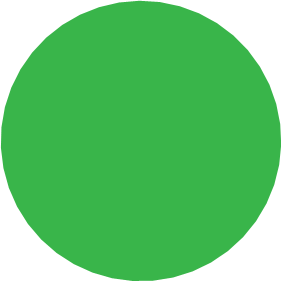SEARCH HOMEMath Central Quandaries & QueriesQuestion from Akino: What do you call a 43-sided polygon and what is its interior angle's total measure?Hi,

I assume you are expecting a regular polygon, one where all the sides are the same length and all the interior angles have the same measure. We have a list of the names of polygons but it only goes up to 20 sides so that's no help. I asked Photoshop to draw a regular polygon with 43 sides and to me it looks like a circle.For a polygon with $n$ sides where $n$ is larger than 6 mathematicians usually call them n-gons. Thus a polygon with 43 sides I would call it a 43-gon.

For the angle measures Walter showed us a nice way to see that the external angle at each vertex of a regular 43-gon is has a measure of $\large \frac{360}{43}$ degrees. What is the measure of the interior angle at each vertex?

PennyMath Central is supported by the University of Regina and The Pacific Institute for the Mathematical Sciences.